Processing ......FreeComputerBooks.com Links to Free Computer, Mathematics, Technical Books all over the World

An Introduction to Quantum Machine Learning for Engineers

• Title: An Introduction to Quantum Machine Learning for Engineers
• Author(s) Osvaldo Simeone
• Publisher: Now Publishers (July 27, 2022); eBook (arxiv.org, 2022)
• Hardcover/Paperback: N/A
• eBook: PDF (240 pages), PostScript. DVI, etc.
• Language: English
• ISBN-10: 1638280584
• ISBN-13: 978-1638280583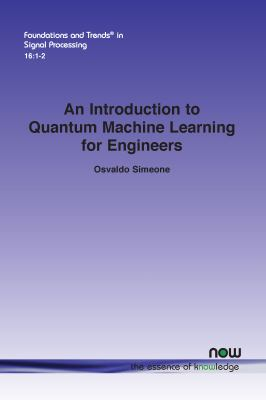Book Description

This monograph provides a self-contained introduction to Quantum Machine Learning for an audience of engineers with a background in probability and linear algebra. It first describes the necessary background, concepts, and tools necessary to describe quantum operations and measurements. Then, it covers parametrized quantum circuits, the variational quantum eigensolver, as well as unsupervised and supervised quantum machine learning formulations.

• Osvaldo Simeone is a Professor of Information Engineering in the Department of Informatics, King's College, London, UK.
Reviews, Ratings, and Recommendations: Related Book Categories: Read and Download Links:Similar Books:
•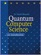Quantum Computer Science: An Introduction (David Mermin)

Quantum Physics has some spectacular applications in computer science. This book is a concise introduction to Quantum Computation, developing the basic elements of this new branch of computational theory without assuming any background in physics.

•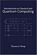Introduction to Classical and Quantum Computing (Tom Wong)

This book is for students who want to learn quantum computing beyond a conceptual level, but who lack advanced training in mathematics. The only prerequisite is trigonometry, and mathematics beyond that will be covered.

•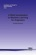A Brief Introduction to Machine Learning for Engineers

This book aims at providing an introduction to key concepts, algorithms, and theoretical frameworks in machine learning, including supervised and unsupervised learning, statistical learning theory, probabilistic graphical models and approximate inference.

•The Hundred-Page Machine Learning Book (Andriy Burkov)

Everything you really need to know in Machine Learning in a hundred pages! This book provides a great practical guide to get started and execute on ML within a few days without necessarily knowing much about ML apriori.

•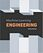Machine Learning Engineering (Andriy Burkov)

The most complete applied AI book out there. It is filled with best practices and design patterns of building reliable machine learning solutions that scale. It embraces the most important thing you need to know about machine learning: mistakes are possible.

•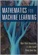Mathematics for Machine Learning (Marc P. Deisenroth, et al.)

This self contained textbook bridges the gap between mathematical and machine learning texts, introducing the mathematical concepts with a minimum of prerequisites. It provides a beautiful exposition of the mathematics underpinning modern machine learning.

•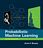Probabilistic Machine Learning: An Introduction (Kevin Murphy)

This book is a comprehensive introduction to machine learning that uses probabilistic models and inference as a unifying approach. It is written in an informal, accessible style, complete with pseudo-code for the most important algorithms.

•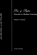Pen and Paper Exercises in Machine Learning (Michael Gutmann)

This is a collection of (mostly) pen-and-paper exercises in machine learning. The author assumes basic calculus, linear algebra, probability and statistics but no prior exposure to machine learning.

•Interpretable Machine Learning: Black Box Models Explainable

This book explains to you how to make (supervised) machine learning models interpretable. The book focuses on machine learning models for tabular data (also called relational or structured data) and less on computer vision and NLP tasks.

•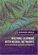Machine Learning with Neural Networks (Bernhard Mehlig)

This modern and self-contained book offers a clear and accessible introduction to the important topic of machine learning with neural networks. It provides comprehensive coverage of neural networks, their evolution, their structure, their applications, etc.

•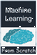Machine Learning from Scratch (Danny Friedman)

This book covers the building blocks of the most common methods in machine learning. This set of methods is like a toolbox for machine learning engineers. Those entering the field of machine learning should feel comfortable with this toolbox.

•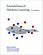Foundations of Machine Learning (Mehryar Mohri, et al)

This book is a general introduction to machine learning. It covers fundamental modern topics in machine learning while providing the theoretical basis and conceptual tools needed for the discussion and justification of algorithms.

•Understanding Machine Learning: From Theory to Algorithms

This book explains the principles behind the automated learning approach and the considerations underlying its usage. It provides an extensive theoretical account of the fundamental ideas underlying machine learning and the mathematical derivations that transform these principles into practical algorithms.

•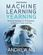Machine Learning Yearning (Andrew Ng)

In this book you will learn how to align on ML strategies in a team setting, as well as how to set up development (dev) sets and test sets. After finishing this book, you will have a deep understanding of how to set technical direction for a machine learning project.

Book Categories
 :All CategoriesRecent BooksMiscellaneous BooksComputer LanguagesComputer ScienceData Science/DatabasesElectrical EngineeringJava and Java EE (J2EE)Linux and UnixMathematicsMicrosoft and .NETMobile ComputingNetworking and CommunicationsSoftware EngineeringSpecial TopicsWeb Programming
Other Categories